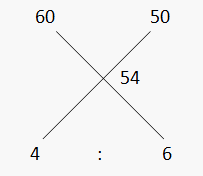# MIXTURE and ALLIGATION: Theory and Basics

###### Understanding Alligation and Mixture
Khal Drogo, the owner of a departmental store, bought two different kinds of sugar, one costing Rs. 60/- and the other Rs. 50/-. He bought different quantities of both and mixed them. After mixing, he could recall that he bought a total of 100 kg for Rs. 5400. Now, as he spent Rs. 5400 for buying 100 kg, the average price per kg is 5400/100 = Rs. 54/-. Now in order to decrease his average price, Khal Drogo wants to buy more quantity of sugar worth Rs. 50. But the problem is that he does not remember the earlier quantities bought at each price.  This made his wife, Khaleesi very sad. Let us find a way to help Khal Drogo find a solution and make Khaleesi happy.
Now to find the answer, we can make the two equations like
x + y = 100; Total sugar purchased
60x + 50y = 5400; Total expenditure
Solving these equations we can find the value of x to be 40 and y to be 60. There is another way to find the quantity bought at each price, which states to write the two extreme prices at the top and their average price in the middle and then find the differences as explained below ;That implies that he has purchased those in the ratio of 4 : 6, which is 2 : 3. For finding the answer, you need to break 100 kg given in this ratio to get the answer as 40 kg and 60 kg.
###### Rule of Alligation:
Now here are some important points, which are to be kept in mind before applying alligation method.
• While comparing the extreme values and average price, you have to take the ‘difference’ between the two values i.e. it is always positive, you have to subtract the smaller value from the larger value.
• The difference so obtained is to be written in the same directions as the lines of the cross are indicating. The difference of the price on the left and the average price will be going towards the right-hand side and the vice versa.
• The ratio appearing will stand for the price below which it is visible, i.e. in this example the ratio of 4: 6 is for sugar worth Rs. 60 and Rs. 50 respectively.
• This technique is applicable in all the cases, where you have two extreme values and their average price. So in the questions from the chapters like percentage, profit and loss, simple interest and average, the technique of mixture and alligation is applicable.
The rule of alligation states "When different quantities of different ingredients are mixed together to produce a mixture of a mean value, the ratio of their quantities is inversely proportional to the differences in their cost from the mean value."